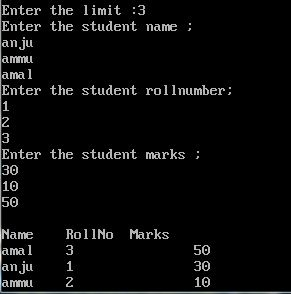UncleCoder.com

UncleCoder.com

Free programming examples and instructions

# C program to sort a set of data using structure

## C program for how to sort a set data using structure

by Krishna

Posted on 27 Jun 2018 Category: C Views: 869

C program to sort a set of student  data  using  structure

In the last article, we have seen the definition of a  structure, its syntax and difference between array and structure. Now we will discuss about C programs based on structure.

Here is the sample C code to sort a list of student data based on the marks using structure.

Here a structure named student is defined with elements name, roll number, and marks.

``````#include <stdio.h>
#include <conio.h>
#include <string.h>
struct student                         //declare structure
{
char name;
int roll,marks;
};
void main()
{
struct student s;
struct student temp;
int i=0,j=0,n;
printf(“Enter the limit :\n”);
scanf(“%d”,&n);
printf(“Enter the student name :\n”);
for(i=0;i<n;i++)
{
gets(s[i].name);
}
printf(“Enter the student rollnumber :\n”);
for(i=0;i<n;i++)
{
scanf(“%d”,&s[i].roll);
}
printf(“Enter the student mark :\n”);
for(i=0;i<n;i++)
{
scanf(“%d”,&s[i].mark);
}
printf(“\nName\tRollnumber\tMarks”);
for(i=0;i<n;i++)
{
for(j=0;j<n-1-i;j++)
{
if((s[i].mark)<(s[j+1].mark))    //sorting based on marks
{
temp=s[j];
s[j]=s[j+1];
s[j+1]=temp;
}
}
}
for(i=0;i<n;i++)
{
printf(“\n%s\t\t%d\t\t%d”,s[i].name,s[i].roll,s[i].mark”);
}
getch();
}
``````

OUTPUT